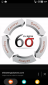1 post / 0 newThe solid compound shaft, made of three different materials, carries the two torques shown. (a) Calculate the maximum shear stress in each material. (b) Find the angle of rotation of the free end of the shaft. The shear moduli are 28 GPa for aluminium, 83 GPa for steel and 35 GPa for bronze.• Mathematics inside the configured delimiters is rendered by MathJax. The default math delimiters are $$...$$ and $...$ for displayed mathematics, and $...$ and $...$ for in-line mathematics.